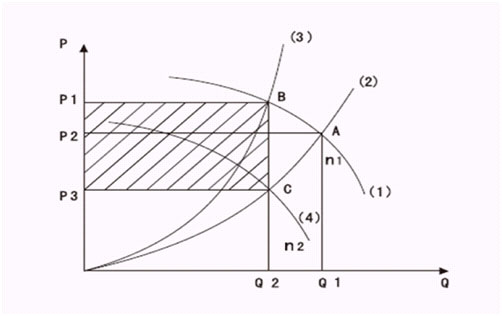﻿ Frequency inverter for fans for energy saving

Details

HomeApplicationsFrequency inverter for fans for energy saving

# Frequency inverter for fans for energy saving

As the fan's static pressure Pst = 0, its energy saving principle can be represented by the diagramIn the diagram, the curve (1) represents the wind pressure and wind quantity characteristics curve (P-Q) at rated speed N1. The curve (2) is the pipe network resistance characteristics curve when the air valve is widely open. The intersection A of the curve (1) and (2) indicates the point at which the motor runs with the highest efficiency. At this time, the wind pressure is assumed as P2, the wind quantity is Q1, and the axis power N1=k*Q1*P2, which can be represented by the area of AP2OQ1.

On condition that the wind quantity is to be decreased from Q1 to Q2 according to production requirements:
If adjusting the wind quantity by the valve, it will increase resistance of the pipe network, resulting in the pipe network resistance curve to change to the curve (3), and the intersection A change to B. At this time, the wind press is assumed as P1 and wind quantity is Q2, so the axis power N2 = k*Q2*P1, which can be represented by the area of BP1OQ2. Compared with AP2OQ1, it is easy to find out that the axis power decreases just a bit.

When adopting the frequency inverter to conduct speed adjustment, the fan speed will reduce from N1 to N2. As the fan's static pressure Pst=0, conforming to the similarity theorem, the wind pressure and wind quantity characteristics curve(P-Q) at the speed N2 can be represented by the curve (4), which converges the curve (2) at point C. At this time, the wind pressure is assumed as P3 and wind quantity is Q2, so the axis power N3=K*Q2*P3, which can be represented by the area of CP3OQ2. It is easy to draw a conclusion that N3 decreases steeply due to hefty decline of wind pressure.
Compared with the valve control mode, the frequency inverter can help save huge energy. The saved axis power ⊿N=K*(P1-P3)*Q2, which can be represented by the area of P1BCP3.

You may like:High quality frequency inverters for electric motor speed controls in energy-saving solutions.

Category

inverters

Featured

Recent